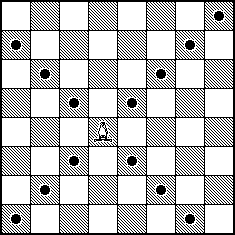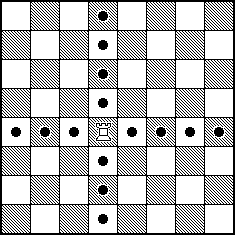## Exercise 1

Just before their last year at Anytown High School, the seniors hold the Senior Year Kick-Off party. Sixty percent of the students attending the party are seniors, and the rest (friends, significant others, siblings, etc.) are juniors (25%), sophomores (10%), and freshmen (5%) Unfortunately, drinking is quite common at this party; 90% of the seniors consume alcohol, so do 80% of the juniors, 50% of the sophomores, and 20% of the freshmen.

(a) If a student at this party is drinking, what is the probability that this student is a senior?

(b) If a student at this party is not drinking, what is the probability that this student is not a senior?

(c) If a student at this party is not a senior, what is the probability that this student is not drinking?

(d) What proportion of the underclassmen (freshmen and sophomores) attending the party consume alcohol?

(e) The school administration discourages the Senior Year Kick-Off party; the principal of AHS announced that any senior attending the party will receive a week of detention. Of course, drinking is also discouraged. Find the proportion of the students at the party who either are seniors, or consume alcohol, or both.

(f) Are events {a student at the party is a senior} and {a student at the party is drinking} independent? Justify your answer. No credit will be given without proper justification.

(g) Are events {a student at the party is a junior} and {a student at the party is drinking} independent? Justify your answer. No credit will be given without proper justification.

## Exercise 2

A bishop is placed at random (with equal chance) on a chess board (8 x 8). A king of the opposing color is placed at random (with equal chance) on one of the remaining squares. What is the probability that the king is under attack from the bishop?Bishop: possible attacks.

Hint: Placed anywhere on a chess board, a rook attacks 14 squares out of the remaining 63.Rook: possible attacks.

$P(\text{ K is under attack }) = \frac{14}{63}$

# Exercise 3

You are given that $$P(A) = 0.5$$ and $$P(A \cup B) = 0.7$$. Student 1 assumes that $$A$$ and $$B$$ are independent and calculates $$P(B)$$ based on that assumption. Student 2 assumes that $$A$$ and $$B$$ are mutually exclusive and calculates $$P(B)$$ based on that assumption. Find the absolute difference between the two calculations.

# Exercise 4

Alex and David agreed to play a series of tennis games (as many as needed) until one of them wins two games in a row. Alex will serve in the first game, then the serve would alternate game by game between Alex and David. David is a better tennis player; Alex has a 50% chance of winning a game on his serve and only a 20% chance of winning a game if David serves. Assume that all games are independent. Find the probability that Alex is the first one to win two games in a row.